# Iteration

## How iteration is done

The mechanism used to perform iteration described in the two papers on Full Metal Jacket is now obsolete, though it will make a limited comeback when we examine macros. Iteration is now performed in Full Metal Jacket by means of feedback. This, however, poses a problem: how do we get feedback in an acyclic graph?

The answer is that while the visible graphs are acyclic, the underlying graphs through which the data really flow are cyclic whenever iteration is used.

Iteration uses two special linked vertices called `start+` and `repeat+`. The `start+` vertex has an equal number of inputs and outputs. When a value is received on every input of `start+`, each value is output on the corresponding output. The `repeat+` vertex has the same number of inputs as `start+`, each of which has the same type. An edge connected to an input on `repeat+` really sends its values to the corresponding input on `start+`.

So `repeat+` is just an extension of `start+`, and has no independent existence. Its inputs can be treated as the inputs of the `start+` vertex to which it is linked.

## Counting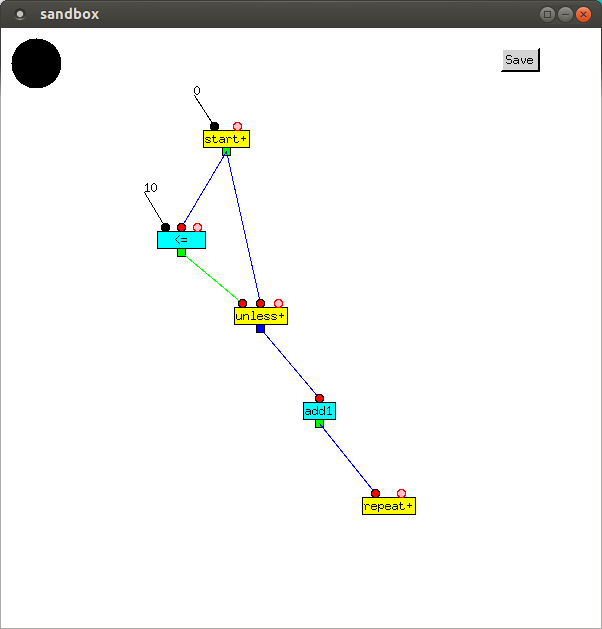`start+` repeatedly outputs a value, starting with 0, to `=` and `unless+`. Unless 10 is less than or equal to the value, it is transcribed (see blue output), then incremented by `add1`, and then sent back to the input of `start+`, and another iteration begins.

zeroth iteration:

 Inputs Vertex Outputs `0` `start+` `0` `100` `<=` `NIL` `NIL0` `unless+` `0` `0` `add1` `1` `1` `repeat+`

first iteration:

 Inputs Vertex Outputs `1` `start+` `1` `101` `<=` `NIL` `NIL1` `unless+` `1` `1` `add1` `2` `2` `repeat+`

...

tenth iteration:

 Inputs Vertex Outputs `10` `start+` `10` `1010` `<=` `T` `T10` `unless+` `add1` `repeat+`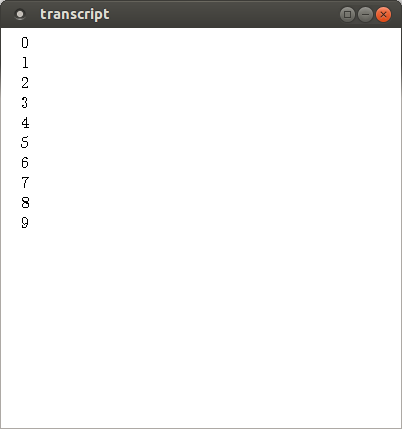## Reading a file and printing its contents

Similary, we can print the contents of a file. Files should really be obsolete: a modern operating system should have different types of objects and the ability to address them as if they were in main memory. Better still, there shouldn't be an operating system. Programming languages should run on the bare metal. But, for now, we're stuck with Unix.

`openFile` takes a file name and a mode, and outputs a channel from which the data in the file can be read. `unless+` has three inputs and two outputs. Unless the end of file has been reached (tested by `EOFp`), the first value output is the second value input, a line of the file; and the second value output is the third value input, the channel through which the file's contents are read.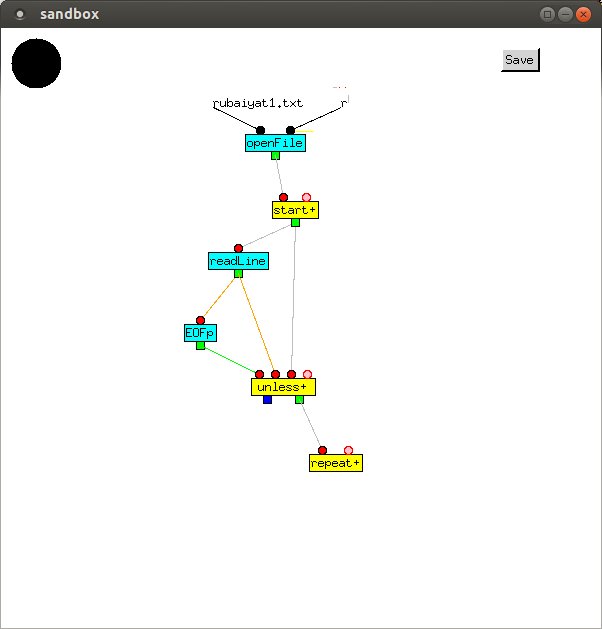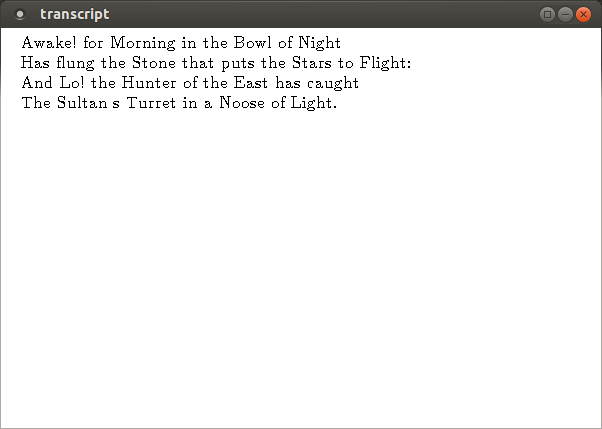## Newton's method

Next, we compose a function containing feedback, which implements Newton's method for calculating the square root of a number. This is done by first approximating the square root, and then repeatedly calculating better values until the differences are small:

```    repeat
a' ← (x / a + a) / 2
until |a' - a| < 0.000001;
return a'
```

where `x` is the number whose square root we want, `a` is the current approximation, and `a'` is the next approximation. The value of `x` remains the same in each iteration, so must be sticky.

The first input is the number we want the square root of, and the second is our initial approximation. We hard-coded the accuracy as `0.000001`, but could, instead, have made it a third input.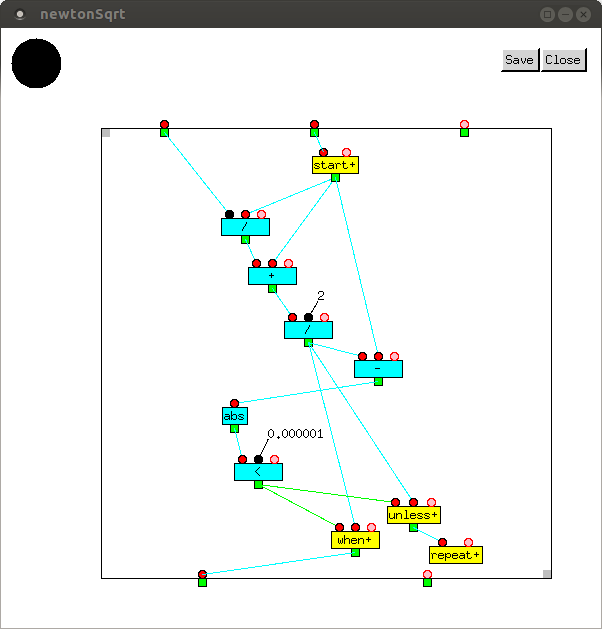First, √10.0 is calculated using the built-in function. Our implementation of Newton's method is correct to the accuracy chosen, even with 1.0 as the first approximation.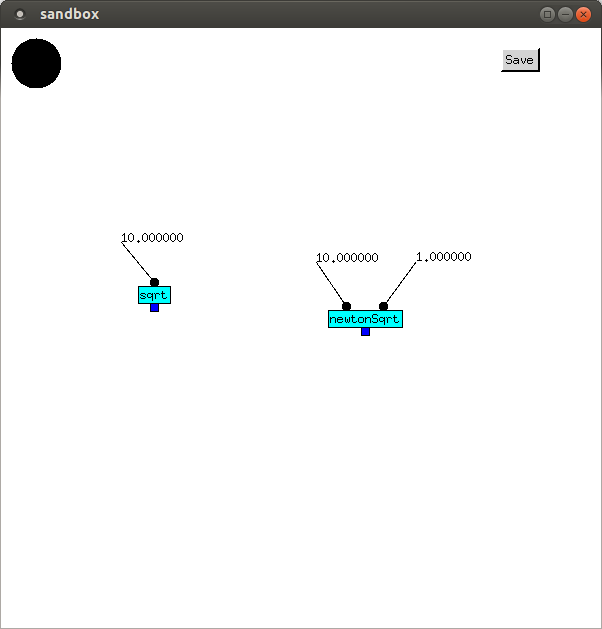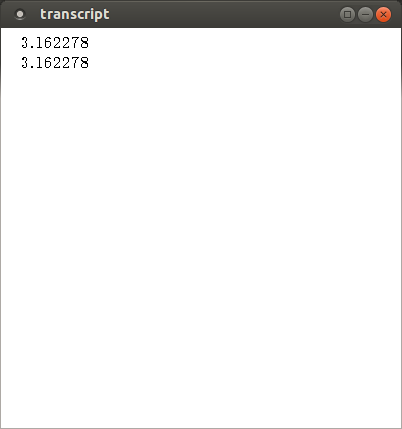## Balancing inputs

It is important that the same number of values arrive at each input of a vertex in any computation. If they do not, a queue will build up, containing the excess values.

During a call,

1. One value is transmitted from each ingate.
2. One value is received on each outgate.
3. If N values are received on each input of `when+` and `unless+`, and A values are sent from each output of `when+`, then N-A values will be sent from each output of `unless+`.
4. One value is received on each input of `start+`, N values are sent from each output, and N-1 values are received on each input of `repeat+`. (NB This is only what appears to happen. When executing, values are really sent directly to `start+`, bypassing `repeat+`.)
5. All other vertices receive N values on each of their inputs, and send N values from their outputs.

The above rules can be applied statically, to verify that the same number of inputs is received on each input of a vertex. If this does not happen, it indicates a bug, even when the function being checked returns correct values.